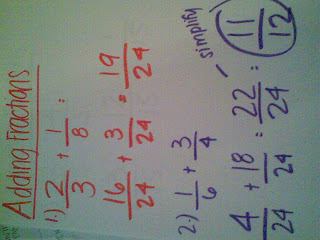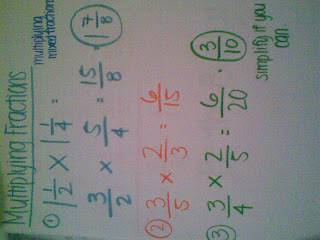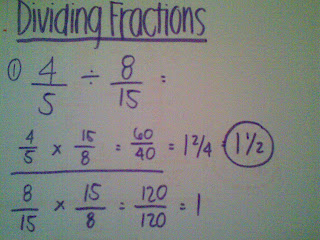### Michelle's Last Fraction Post

Monday, May 11, 2009- First make the denominators the same by multiplying the denominators together, or finding the number that they can both go into.
- Add numerator to numerator and denominator to denominator
- You could simplify if the numerator and denominator are not prime numbers

SUBTRACTING FRACTIONS:- First make the denominators the same by multiplying the denominators together, or finding the number that they can both go into.
- Subtract numerator to numerator and denominator to denominator
- You could simplify if the numerator and denominator are not prime numbers

MULTIPLYING FRACTIONS:Multiplying proper fractions:
- Multiply numerator to numerator and denominator to denominator
- Simplify if answer is not a prime number

Multiplying mixed fractions:
- Convert mixed fractions to improper fractions
- Multiply numerator to numerator and denominator to denominator
- Simplify if answer is not a prime number

DIVIDING FRACTIONS:- First you must find the reciprical. The reciprical is one of the fractions upside down.
- Multiply the numerators and denominators
- Convert the fraction into mixed fractions
- Simplify if answer is not a prime number

Dividing mixed fractions:- Convert mixed fractions into improper fractions
- BE THE BULLY
- Find the reciprical
- Multiply numerator to numerator and denominators to denominators
- Convert answer to mixed fraction

WORD PROBLEMS: